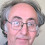## Friday, November 20, 2015

### News about my1965 Proof of Fermat's Last Theorem

More than 50 years ago I made some profound discoveries that I’ve used over the years to explore the world of mathematical physics and the relation between quantum mechanics and consciousness. This adventure began when I developed a proof for the famous mathematical conundrum known as Fermat’s Last Theorem in 1965.
My proof, known as FLT65, has never been refuted. Fermat’s Last Theorem, thought by most to be an abstract number theory theorem, has serious application to quantum physics and It is being currently being reviewed in connection with new research.

This is the first in a series of posts of sections of this paper currently being reviewed by  number of mathematicians and scientists.

CLARIFICATION AND EXPLANATION OF FLT65
A 1965 PROOF OF FERMAT’S LAST THEOREM
By Edward R. Close, PhD

ABSTRACT:
Fermat’s last theorem (FLT) states that no three positive integers X1, Y1, and Z1 can satisfy the equation xn +yn = zn for any integer value of n greater than two. Fermat penned the following statement in Latin in the margin of a book on Diophantine equations in 1637:

Concerning whole numbers, while certain squares can be separated into two squares, it is impossible to separate a cube into two cubes or a fourth power into two fourth powers or, in general any power greater than the second into two powers of like degree. I have discovered a truly marvelous demonstration, which this margin is too narrow to contain.”
-        Pierre de Fermat, circa 1637 

Fermat’s marvelous proof was never found and the theorem remained officially a conjecture without proof until Andrew Wiles published a lengthy treatment in 1995 that was accepted by number theorists as a valid proof . Prior to that, however, Edward R. Close completed a proof in 1965 (FLT65), submitted it to the first of many reviewers in 1966, and published it in 1977 . The Close proof though never refuted, presented difficulties for some reviewers because of unconventional notation, and at least three reviewers have suggested that the difference between applying the division algorithm to algebraic polynomial factors and integer factors of the equation as used in the proof make it questionable and either incomplete or incorrect. This concern is addressed in this paper and shown to be unwarranted: There has been a tendency for reviewers to ignore the uniqueness requirements for the division algorithm stated in FLT65 while looking for mistakes which they assume must be there, for reasons explained in this paper. When everything presented in FLT65 is appropriately considered, the concern over the application of the division algorithm is eliminated, removing the only serious objections to the proof.
______________
In this paper, the author provides a brief description and history of FLT65, followed by presentation and refutation of the objections and supposed counterexamples offered by some reviewers in efforts to invalidate FLT65. Several appendices are attached, including a copy of the original FLT65 proof. The other appendices contain supporting arguments and information confirming that FLT65 was and is, a valid proof, justifying the statement found on the last page of FLT65:

“… we have reached a complete contradiction by assuming X, Y and Z to be integers, and may state that the equation XN + YN = ZN has no solutions in positive integers when N is an integer > 2. And so the proof of Fermat’s last theorem is complete.

-        Edward R. Close, December 18, 1965 

#### 1 comment:

1.'Never been refuted'? It seems Close never accepted our email exchange (sorry the super/subscripts don't copy), which continued for quite some time but made no progress:

On 12 Jan 2014, at 01:19, Edward Close wrote:

I responded
Fine! I’ve done that, and would query this part:

F(Z) = ZN – X1N - Y1N = 0, and thus can be expanded to produce:

F(Z) = (Z-X1)( ZN-1 + ZN-2X1 + ZN-3 X12 +•••+ X1N-1) - (Z-X1)(Z –a )N = 0.

By inspection of this equation, we see that G(Z) is a polynomial factor of F(Z) ...

We can see the error here by using z for the variable and Z for the constant figuring in the Fermat eqn. and I will keep track of the difference by using z and Z as appropriate.

We need an expression for F(z). The definition is F(z) = zN – X1N - Y1N

The problem is that the first part of this factorises into
(z-X1)( zN-1 + zN-2X1 + zN-3 X12 +•••+ X1N-1)

and for the second part, by combining
g(Z)f(Z) = YN (eqn. 3)
g(z) = z - X1
and f(Z) = (Z –a )N

we end up with (Z-X1)(Z –a )N.

Thus the first term of that expanded version of F(z) above involves the variable z, while the second involves the constant Z. So the factorisation of F that you rely on doesn’t apply.

In response Close wrote:

The only advantage I have is: I know FLT is true. Ergo there must be a way around it. When I find it (because I believe I will), I will send it along. Thank you for being interested in seeing it.